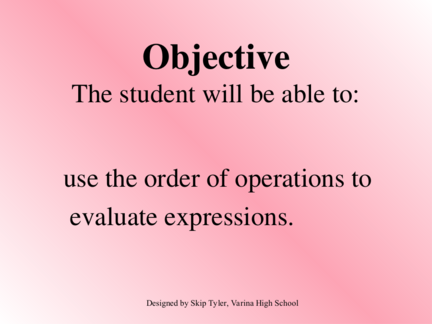# Order of Operations to Evaluate ExpressionsContributed by:Order of operations: Parenthesis, Exponents or powers, Multiply and Divide, Add and Subtract. Simplification of mathematical expressions.
1. Objective
The student will be able to:
use the order of operations to
evaluate expressions.
Designed by Skip Tyler, Varina High School
2. Evaluate 7 + 4 • 3.
You can get 2 different answers
depending on which operation you
did first. We want everyone to get
the same answer so we must follow
the order of operations.
3. Remember the phrase
“Please Excuse My Dear Aunt Sally”
or PEMDAS.
ORDER OF OPERATIONS
1. Parentheses - ( ) or [ ]
2. Exponents or Powers
3. Multiply and Divide (from left to right)
4. Add and Subtract (from left to right)
4. Once again, evaluate 7 + 4  3
and use the order of operations.
= 7 + 12 (Multiply.)
5. Example #1
14 ÷ 7 • 2 - 3
= 2 • 2 - 3 (Divide l/r.)
= 4 - 3 (Multiply.)
= 1 (Subtract.)
6. Example #2
3(3 + 7) 2 ÷ 5
= 3(10) 2 ÷ 5 (parentheses)
= 3(100) ÷ 5 (exponents)
= 300 ÷ 5 (multiplication)
= 60 (division)
7. Example #3
20 - 3 • 6 + 102 + (6 + 1) • 4
= 20 - 3 • 6 + 102 + (7) • 4 (parentheses)
= 20 - 3 • 6 + 100 + (7) • 4 (exponents)
= 20 - 18 + 100 + (7) • 4 (Multiply l/r.)
= 20 - 18 + 100 + 28 (Multiply l/r.)
= 2 + 100 + 28 (Subtract l/r.)
= 102 + 28 (Add l/r.)
8. Which of the following represents
112 + 18 - 33 · 5 in simplified form?
1. -3,236
2. 4
3. 107
4. 16,996
9. Simplify
16 - 2(10 - 3)
1. 2
2. -7
3. 12
4. 98
10. Simplify
24 – 6 · 4 ÷ 2
1. 72
2. 36
3. 12
4. 0
11. Evaluating a Variable Expression
To evaluate a variable expression:
1. substitute the given numbers for each
variable.
2. use order of operations to solve.
12. Example # 4
n + (13 - n)  5 for n = 8
= 8 + (13 - 8)  5 (Substitute.)
= 8+55 (parentheses)
= 8+1 (Divide l/r.)
13. Example # 5
8y - 3x2 + 2n for x = 5, y = 2, n =3
= 8  2 - 3  52 + 2  3 (Substitute.)
= 8  2 - 3  25 + 2  3 (exponents)
= 16 - 3  25 + 2  3 (Multiply l/r.)
= 16 - 75 + 2  3 (Multiply l/r.)
= 16 - 75 + 6 (Multiply l/r.)
= -59 + 6 (Subtract l/r.)
14. What is the value of
-10 – 4x if x = -13?
1. -62
2. -42
3. 42
4. 52
15. What is the value of
5k3 if k = -4?
1. -8000
2. -320
3. -60
4. 320